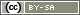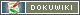This translation is older than the original page and might be outdated. See what has changed.

# statistic()

`mixed statistic(string statistics, array|string variables, mixed option, [boolean AllData])`

The statistic() function can be used to obtain univariate characteristic values from the data set (across all previous interviews).

• Statistic
Which statistic should be determined?
• `'count'` – Count the frequency of the value given as `option`.
• `'percent'` – Percentage of the value specified as `Option`.
• `'frequencies'` – frequencies for all response codes in the dataset (as an array).
• `'crosscount`' – Count the frequency of co-occurrence of two values in two variables. The two variables are to be specified as an array (or separated by a comma), as are their values specified as `option`.
• `'mode`' – most frequently occurring value.
• `'min`' – Smallest value.
• `'max`' – Largest value.
• `'mean`' – Arithmetic mean of the values.
• `'groupmean`' – Arithmetic mean of the values of a subgroup defined by `Option`, specified as a string consisting of variable name and code for the cases to be counted `'AB01=2`'.
• `'filter`' – Specifies which cases to use in further calls to the function `statistic()` (see bottom for details).
• Variables
Specifies for which variable(s) the statistic is to be calculated. The identifiers of the individual variables can be found in the Variables overview. If the statistic requires several variables, these can be specified either as a comma-separated string or as an array.
• Option
Some statistics require or allow a third specification, which is given with this parameter (see below).
• AllData
This optional specification determines that not only the completed interviews but all interviews are included in the statistics.

Important: Only completed interviews are included in the calculation of statistical values if `true` is not explicitly specified for the parameter allData.

Important: Test data from questionnaire development and pretest are only counted if the current interview is also part of the test. If the interview is conducted as part of the regular data collection, `statistic()` only counts data from the regular data collection.

Note: The data from the current interview is not taken into account by `statistic()`.

Note: The use of `statistic()` may be inefficient. If the questionnaire has to search the whole dataset several times in several `statistic()` calls, a warning will be displayed first. If there are more than 10 computationally intensive calls, `statistic()` will no longer return results. Use `statistic('load', …)` to load the data in advance to avoid this problem.

Tip: The function `statistic()` can be used to close the questionnaire after reaching a predefined quota (Quota) and either display a message to further participants or redirect them to the quota stop link of a panel provider.

Tip: If you do not want to count all completed interviews (e.g. if dropouts were redirected to another page using `redirect()`), it makes sense to copy the variable to be counted further back in the questionnaire into an Internal Variables.

## Frequency Count I

As a third argument in a frequency count (`'count`'), you can specify for which value you want to determine the frequency. If you do not specify a third value, the number of valid answers is output. Missing data are not counted.

For example, if you have a selection for the gender (1=female, 2=male, -9=not specified), you can determine the number of women by specifying the third value `1`:

```\$countWomen = statistic('count', 'SD01', 1); // frequency women (1)
\$countMen = statistic('count', 'SD01', 2); // frequency men (2)
\$countDone = statistic('count', 'SD01'); // number of valid dates
\$countAll = statistic('count', 'SD01', false, true); // All records
html('
<p>So far in this survey '.\$countAll.' Persons
have provided information about their gender, but the
interview was completed only in '.\$countDone.' Cases.</p>
<p>The completed interviews include '.
\$countWomen.' Women and '.
\$countMen.' men.</p>
');
question('SD01'); // Question about one's own gender```

## Frequency Count II

The `'frequencies'` statistic returns all possible values with one call.

Note: Note that the array only contains entries for the response codes whose responses are present at least once in the data set. Therefore, check whether the array key is present. This is possible, for example, with the `??` operator.

```\$freq = statistic('frequencies', 'SD01'); // frequencies
\$numberWomen = (\$freq ?? 0);
\$numberMen = (\$freq ?? 0);
html('
<p>The completed interviews include '.
\$numberWomen.' Women and '.
\$numberMen.' men.</p>
');
question('SD01'); // Question about one's own gender```

## Multivariate frequency

With the `'crosscount`' statistic, one can count (as in a crosstab) the cases where several variables apply.

Instead of a single variable, specify 2 or more variables as an array or separated by a comma (`,`). The third parameter `option` is used to specify which values are counted for each variable. Only cases are counted that have specified the first value for the first variable, the second value for the second variable, and so on.

```\$nYoungFemale = statistic('crosscount', 'SD01,SD02', '2,1'); // Variables and values as comma list ...
\$nGrownFemale = statistic('crosscount', array('SD01','SD02'), array(2,2)); // ... or as arrays
html('
<p>So far in this survey '.\$nYoungFemale.' People
have indicated that they are female and in age group 1 (up to 18 years).
'.\$nGrownFemale.' Females indicated an age of 19 years or older.</p>
');
question('SD01'); // Question about one's own gender
question('SD02'); // Question about one's own age```

## Valid percent

The output is the share of a value in all valid entries. The third argument must be the value to be counted.

```\$proportionWomen = statistic('percent', 'SD01', 1); // proportion of women.
html('
<p>So far in this survey '.
\$proportionWomen.' Women participated.</p>
');
question('SD01'); // Frage nach dem eigenen Geschlecht```

## Mode, most frequently specified value

Returns the value that has been selected most often so far. If multiple values have been selected equally often, then they are returned separated by a comma.

As a third argument (in this case of type Boolean) you can specify whether invalid values (no response, etc.) are also counted.

```\$modus = statistic('mode', 'AB01_02', true);
\$modi = explode(',', \$modus); // Separate multiple values
if (count(\$modi) > 1) {
// Several most frequently mentioned values
html('
<p>Several answers were chosen equally often.</p>
');
} else {
// Texts of the answer options (statistic() returns only the numeric code)
\$text = getValuetext('AB01_02', \$modus);
html('
<p>The most common answer to this question was: '.\$text.'.</p>
');
}```

## Min, max and mean value of the valid entries

The statistics `'min`', `'mean`' and `'max`' will only calculate a correct value if numeric values are available for the question. In the case of a text entry, entries that are not numbers will be ignored – unless it is specified as a third parameter (`true`) that invalid values should also be included in the statistics.

If there are no valid values so far, 0 is returned for `'mean`', for `'min`' and `'max`' the value `false` is returned.

```\$min = statistic('min', 'BB01_03');
\$max = statistic('max', 'BB01_03');
\$mean = statistic('mean', 'BB01_03');
html('
<p>Participants have given the program an
Average rating of '.\$mean.'</p>.
<p>Ratings range from '.\$min.' to '.\$max.'</p>
');```

## Evaluate partial data sets

By means of `statistic('filter', …)` a filter can be set which will be applied to all further calls of `statistic()`. As a second parameter (optional) Variables can be specified for acceleration, which are needed in subsequent calls.

The number of cases that match the filter is returned. The fourth parameter AllData only affects the return value, but not the further counting.

```// Statistics on female participants only (SD02 = 1)
// The RT variables are loaded immediately to reduce latencies
\$n = statistic('filter', array('RT02_01', 'RT02_02', 'RT02_03'), 'SD02==1');
// Mean of ratings (women only)
\$mean1 = statistic('mean', 'RT02_01');
\$mean2 = statistic('mean', 'RT02_02');
\$mean3 = statistic('mean', 'RT02_03');```

The filter allows common comparison operators (`>`, `>=`, `<`, `<=`, `!=`, `==`), parentheses and and Boolean operators (`AND`, `&&`, `OR`, `||`, `NOT`, `!`).

Note: Comparisons are only possible between one variable and a constant value (a number or a string) at a time, e.g. `SD02==2`, comparisons between two variables (`SD03>SD04`) are not supported.

```// Statistics only on female participants (SD02 = 1) aged 35 and over (SD03 >= 35).
\$n = statistic('filter', false, '(SD02==1) AND (SD03 >= 35)');```

Besides the variable names, `QUESTNNR`, `CASE` and `LANGUAGE` can be used for the filter.

```// Statistics only on female participants (SD02 = 1) aged 35 years and older (SD03 >= 35) in the German language version.
\$n = statistic('filter', false, '(SD02==1) AND (SD03 >= 35) AND (LANGUAGE == "ger")');```